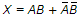# Digital Electronics - Combinational Logic Circuits

### Exercise :: Combinational Logic Circuits - True or False

26.

The Boolean equation of the exclusive-NOR function is.

 A. True B. False

Explanation:

No answer description available for this question. Let us discuss.

27.

To implement the full-adder sum functions, two exclusive-OR gates can be used.

 A. True B. False

Explanation:

No answer description available for this question. Let us discuss.

28.

The input at the 1, 2, 4, 8 inputs to a 4-line to 16-line decoder with active-low outputs is 1110. As a result, output line 7 is driven LOW.

 A. True B. False

Explanation:

No answer description available for this question. Let us discuss.

29.

When decisions demand two possible actions, the IF/THEN/ELSE control structure is used.

 A. True B. False

Explanation:

No answer description available for this question. Let us discuss.

30.

TTL stands for transistor-technology-logic.

 A. True B. False